# What you will need

1. Working and up-to-date installations of R and RStudio
3. An internet connection
4. Optional: A healthy source of caffeine

# Summary

In this section we will dive into R. We start by installing and loading three useful packages (dplyr, haven, readr, and pacman). We then load two datasets and begin summarizing and manipulating them. Finally, we’ll make our first plots.

# Packages in R

Open RStudio. Make sure you are running the most recent versions of R and RStudio.1

While the base R installation helpful/powerful, R’s true potential comes from combining the core installation with the many, many packages generated through (open-source) collaboration (see CRAN’s list of packages2).

## Installing packages

You can check which packages are currently installed by using the function installed.packages(). Ready to run your first function? Type installed.packages() into the R console and hit return. That’s a lot of information. Don’t worry about it for now.

Alternatively (and more easily), you can check the Packages tab inside of RStudio.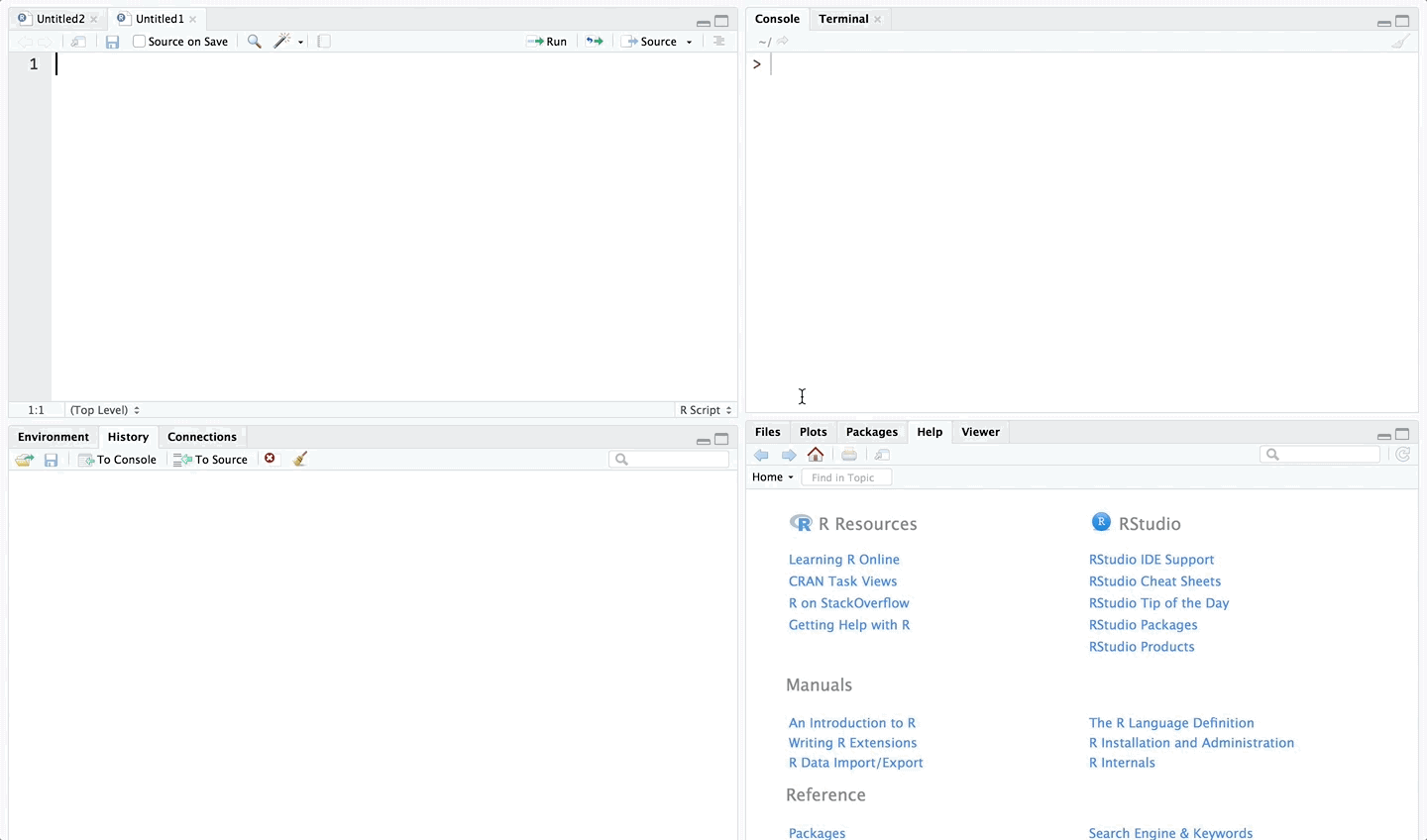The checked boxes denote the packages that are currently loaded.

Now, let’s install a few packages that will prove useful this semester. We will use the creatively named install.packages() function. You just need to give the function that name(s) of the package(s) you want to install.

# Install the package named "dplyr"
install.packages("dplyr")
# Install the packages named "haven" and "readr"
install.packages(c("haven", "readr"))

A few things to notice here:

1. You need an internet connection.
2. The name of the function install.packages() is plural regardless of the number of packages.
3. Each package’s name is surrounded by quotes (e.g., "haven"). These quotation marks are how R knows the difference between characters/strings and objects (objects hold values). R generally does not care whether you use single quote ('') or double quotes (""), but you should be consistent. If you ask me, I say double quotes.
4. We can create a vector of packages using the combine function c(). Example: c(1, 2, 3) is a three-element vector whose elements are 1, 2, and 3. Similarly, c("haven", "readr") is a two-element vector whose elements are the "haven" and "readr". Vectors are a big deal in R.
5. The hashtag (#) is the symbol that creates comments in R.

To check that the installations were successful, we will load the packages that we installed above.

R uses the library() function to load a package (we give the name of the package as the argument to the function).3

library(dplyr)
##
## Attaching package: 'dplyr'
## The following objects are masked from 'package:stats':
##
##     filter, lag
## The following objects are masked from 'package:base':
##
##     intersect, setdiff, setequal, union
library(haven)
library(readr)

Notice that we did not need to call the packages with quotations around their names (though it would still work).

## Package management with pacman

Let’s install another package: the pacman package.

# Install the 'pacman' package
install.packages("pacman")

# Load the 'pacman' package
library(pacman)

In addition to an excellent name, the pacman package is very meta: it’s a package to manage packages. You can use it to install, load, unload, and update your R packages. Plus it really streamlines your code. Rather than having to type library(...) every time you want to load a package, you can use pacman’s handy p_load() function. For instance,

library(dplyr)
library(haven)
library(readr)

becomes

p_load(dplyr, haven, readr)

Another great feature of p_load(): if you try to load a package that is not installed on your machine, p_load() install the package for you, rather than throwing an error. For instance, let’s install and load one final package named ggplot2.

p_load(ggplot2)

1. The path of the data file (where the data exist on your computer)
2. The name of the data file
3. The proper function for the type of dataset (e.g., we use different functions for .csv and .dta files)

R wants file paths as character vectors (i.e., the file path surrounded by quotations). For example, "/Users/edwardarubin/Dropbox/Teaching/ARE212" is the path to my folder for this course (on my computer).4 5

To change the directory in R, use the setwd(). For instance, to change R’s directory to my course folder

setwd("/Users/edwardarubin/Dropbox/Teaching/ARE212")

To find R’s current working directory, simply type getwd():

getwd()
##  "/Users/edwardarubin/Dropbox/Teaching/ARE212"

To navigate your directory, you can combine setwd() with similar commands as you would in Linux, i.e.,

1. .. moves up one level
2. ~ returns to your home directory
3. You can use full paths (/Users/edwardarubin/Dropbox/Teaching/ARE212/Section01) or paths that begin in your current location (Section01)
# The current directory
getwd()
##  "/Users/edwardarubin/Dropbox/Teaching/ARE212"
# Move up one level
setwd("..")
# Check the directory now
getwd()
##  "/Users/edwardarubin/Dropbox/Teaching"
# Go home
setwd("~")
# Check the new working directory
getwd()
##  "/Users/edwardarubin"
# Now return to my ARE212 folder
setwd("Dropbox/Teaching/ARE212")
# Check the working directory
getwd()
##  "/Users/edwardarubin/Dropbox/Teaching/ARE212"

There are a few ways to deal with file paths and directories. One common way is to use setwd() at the top of your script (or invoke the function whenever you need to change directories).

I find clearer to store the paths that I will use—at the start of my R script, I define the paths relevant to the files I use within the script. I prefer this method because it allows me to quickly update paths and easily access subfolders.

# The path to my ARE 212 folder (ARE212)
dir_class <- "/Users/edwardarubin/Dropbox/Teaching/ARE212/"
# The path to my section 1 folder (Section01), which is inside my ARE 212 folder
dir_section1 <- paste0(dir_class, "Section01/")

Notice the use of the paste0() function here: we paste together the value of the object dir_class and the string "Section01". The function paste0(), by default, pastes without any spaces in between the objects. The function paste() defaults to including a single space (you can feed the functions additional parameters to change these behaviors).

As a quick example:

# Default use of paste0()
paste0(1, 2, 3)
##  "123"
# Default use of paste()
paste(1, 2, 3)
##  "1 2 3"
# Setting the separation parameter to " " (the default)
paste(1, 2, 3, sep = " ")
##  "1 2 3"
# Changing the separation parameter to "+"
paste(1, 2, 3, sep = "+")
##  "1+2+3"

What do I mean by object? R holds the characters "/Users/edwardarubin/Dropbox/Teaching/ARE212/" in its memory and assigns to them (the object) the name dir_class.

# The object 'dir_class'
dir_class
##  "/Users/edwardarubin/Dropbox/Teaching/ARE212/"
# The character vector
"Section01/"
##  "Section01/"
# Paste them together
paste0(dir_class, "Section01/")
##  "/Users/edwardarubin/Dropbox/Teaching/ARE212/Section01/"

Finally, notice that RStudio assists you with completing file paths (begin typing and press tab). This completion can be super useful.

Example: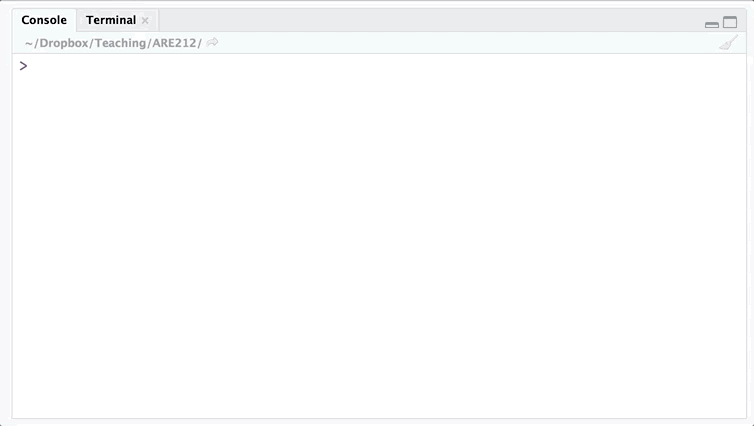Nice, right?

## dir()

The function dir() allows you to see contents of a folder. dir() can help when you forget the name of the file you want. To see the contents of a folder, give the folder’s file path to dir():

# Look inside my ARE212 folder (dir_class stores the path)
dir(dir_class)
##    "_site.yml"             "analyticstracking.php"
##    "contact.html"          "contact.Rmd"
##    "courseInfo.html"       "courseInfo.Rmd"
##    "footer.html"           "futureNotes.html"
##    "futureNotes.Rmd"       "htmlScript.R"
##   "Images"                "index.html"
##   "index.Rmd"             "LatexKnitr"
##   "latexKnitr_files"      "latexKnitr.html"
##   "latexKnitr.Rmd"        "notes.html"
##   "notes.Rmd"             "Papers"
##   "resources.Rmd"         "Section00"
##   "section00.html"        "section00.Rmd"
##   "Section01"             "section01_files"
##   "section01.html"        "section01.Rmd"
##   "Section01.zip"         "Section02"
##   "section02.html"        "section02.Rmd"
##   "Section02.zip"         "Section03"
##   "section03_cache"       "section03_files"
##   "section03.html"        "section03.Rmd"
##   "Section03.zip"         "Section04"
##   "section04_cache"       "section04_files"
##   "section04.html"        "section04.Rmd"
##   "Section04.zip"         "Section05"
##   "section05_cache"       "section05_files"
##   "section05.html"        "section05.Rmd"
##   "Section05.zip"         "Section06"
##   "section06_cache"       "section06_files"
##   "section06.html"        "section06.Rmd"
##   "Section06.zip"         "Section07"
##   "section07_cache"       "section07_files"
##   "section07.html"        "section07.Rmd"
##   "Section07.zip"         "Section08"
##   "section08_cache"       "section08_files"
##   "section08.html"        "section08.Rmd"
##   "Section08.zip"         "Section09"
##   "section09_files"       "section09.html"
##   "section09.Rmd"         "Section09.zip"
##   "Section10"             "section10_files"
##   "section10.html"        "section10.Rmd"
##   "Section10.zip"         "Section11"
##   "section11_cache"       "section11_files"
##   "section11.html"        "section11.Rmd"
##   "Section11.zip"         "Section12"
##   "section12_cache"       "section12_files"
##   "section12.html"        "section12.Rmd"
##   "Section12.zip"         "Section13"
##   "section13_cache"       "section13_files"
##   "section13.html"        "section13.Rmd"
##  "Section13.zip"         "section14_cache"
##  "section14_files"       "section14.html"
##  "section14.Rmd"         "section15.html"
##  "section15.Rmd"         "site_libs"
##  "Spring2017"            "syllabi.html"
##  "syllabi.Rmd"           "syllabus2017.pdf"
##  "syllabus2018.pdf"      "TODO.html"
##  "TODO.md"               "update.bash"
# Look inside my section 1 folder (dir_section1 stores the path)
dir(dir_section1)
##  "auto.csv"      "auto.dta"      "README.md"     "section01.pdf"
##  "section01.R"

You can see there are a few files of interest in the section 1 folder—specifically, auto.csv and auto.dta.6

Recall that dir_section1 is an object that holds a value representing a file path, i.e.,

dir_section1
##  "/Users/edwardarubin/Dropbox/Teaching/ARE212/Section01/"

Notice that we get the same result if we feed dir() object’s name or its value, since R is evaluating the object:

# The object
dir(dir_section1)
##  "auto.csv"      "auto.dta"      "README.md"     "section01.pdf"
##  "section01.R"
# The object's value
dir("/Users/edwardarubin/Dropbox/Teaching/ARE212/Section01/")
##  "auto.csv"      "auto.dta"      "README.md"     "section01.pdf"
##  "section01.R"

There are a lot of ways to load (data) files in R. In this class, we will mostly stick to the packages readr and haven—in addition to R’s base functions. The readr package offers functions for mostly for reading delimited data files like CSVs, TSVs, and fixed-width files. The haven package offers functions for reading data files outputted from other statistical software like Stata, SPSS (or PSPP), and SAS. R also has its own file types (i.e., .rds and .rdata). Eventually, we will also talk about loading spatial data (e.g., shapefiles and various rasters).

Let’s start by reading the data stored in the auto.dta file. For this task, we’ll use the read_dta() funtion from the haven package. The read_dta() function needs only one argument: the file (including the path necessary to reach the file).

1. Press tab (in RStudio) after typing the function’s name.
2. Type a question mark and the function’s name into the console, e.g., ?read_dta (and then hit return).

Example: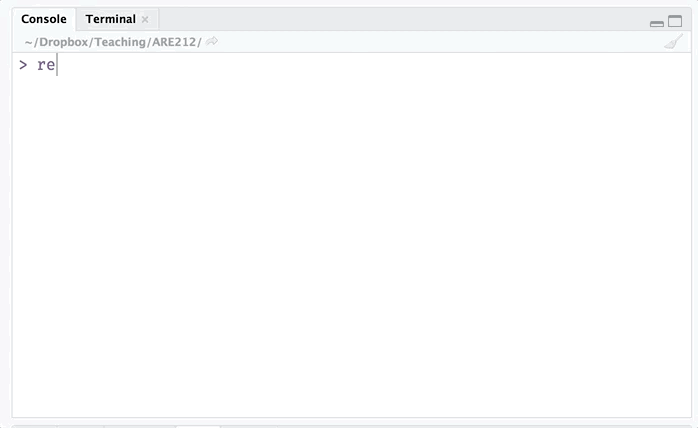Enough talk. Let’s finally load the file.

# Load the .dta file
car_data <- read_dta(paste0(dir_section1, "auto.dta"))

The <- operator is central to everything you do in R. It assigns the value(s) on the right-hand side of the arrow to the name on the left-hand side. When reading R code aloud, people often replace the arrow with “gets”. The main thing to understand is that the contents of “auto.dta” are now assigned to the name car_data. To see this, simply type the name into the console (a bad idea with really big datasets, but this dataset is not big).

car_data
## # A tibble: 74 x 12
##    make           price   mpg rep78 headroom trunk weight length  turn
##    <chr>          <dbl> <dbl> <dbl>    <dbl> <dbl>  <dbl>  <dbl> <dbl>
##  1 AMC Concord    4099.   22.    3.     2.50   11.  2930.   186.   40.
##  2 AMC Pacer      4749.   17.    3.     3.00   11.  3350.   173.   40.
##  3 AMC Spirit     3799.   22.   NA      3.00   12.  2640.   168.   35.
##  4 Buick Century  4816.   20.    3.     4.50   16.  3250.   196.   40.
##  5 Buick Electra  7827.   15.    4.     4.00   20.  4080.   222.   43.
##  6 Buick LeSabre  5788.   18.    3.     4.00   21.  3670.   218.   43.
##  7 Buick Opel     4453.   26.   NA      3.00   10.  2230.   170.   34.
##  8 Buick Regal    5189.   20.    3.     2.00   16.  3280.   200.   42.
##  9 Buick Riviera 10372.   16.    3.     3.50   17.  3880.   207.   43.
## 10 Buick Skylark  4082.   19.    3.     3.50   13.  3400.   200.   42.
## # ... with 64 more rows, and 3 more variables: displacement <dbl>,
## #   gear_ratio <dbl>, foreign <dbl+lbl>

If we instead had a CSV file—which we do—we could use the function read_csv() from the package readr to load the file.7

# Load the .csv file
car_data <- read_csv(paste0(dir_section1, "auto.csv"))
## Parsed with column specification:
## cols(
##   make = col_character(),
##   price = col_double(),
##   mpg = col_double(),
##   rep78 = col_double(),
##   trunk = col_double(),
##   weight = col_double(),
##   length = col_double(),
##   turn = col_double(),
##   displacement = col_double(),
##   gear_ratio = col_double(),
##   foreign = col_integer()
## )
# See that it looks the same as above
car_data
## # A tibble: 74 x 12
##    make           price   mpg rep78 headroom trunk weight length  turn
##    <chr>          <dbl> <dbl> <dbl>    <dbl> <dbl>  <dbl>  <dbl> <dbl>
##  1 AMC Concord    4099.   22.    3.     2.50   11.  2930.   186.   40.
##  2 AMC Pacer      4749.   17.    3.     3.00   11.  3350.   173.   40.
##  3 AMC Spirit     3799.   22.  NaN      3.00   12.  2640.   168.   35.
##  4 Buick Century  4816.   20.    3.     4.50   16.  3250.   196.   40.
##  5 Buick Electra  7827.   15.    4.     4.00   20.  4080.   222.   43.
##  6 Buick LeSabre  5788.   18.    3.     4.00   21.  3670.   218.   43.
##  7 Buick Opel     4453.   26.  NaN      3.00   10.  2230.   170.   34.
##  8 Buick Regal    5189.   20.    3.     2.00   16.  3280.   200.   42.
##  9 Buick Riviera 10372.   16.    3.     3.50   17.  3880.   207.   43.
## 10 Buick Skylark  4082.   19.    3.     3.50   13.  3400.   200.   42.
## # ... with 64 more rows, and 3 more variables: displacement <dbl>,
## #   gear_ratio <dbl>, foreign <int>

Note that you do not have to paste the directory onto the file name if you are already in the file’s directory (R reasonably defaults to looking in the current directory). In my case, I just need to tell R to go to the Section01 folder, where my auto.csv file lives.

read_csv("Section01/auto.csv")
## Parsed with column specification:
## cols(
##   make = col_character(),
##   price = col_double(),
##   mpg = col_double(),
##   rep78 = col_double(),
##   trunk = col_double(),
##   weight = col_double(),
##   length = col_double(),
##   turn = col_double(),
##   displacement = col_double(),
##   gear_ratio = col_double(),
##   foreign = col_integer()
## )
## # A tibble: 74 x 12
##    make           price   mpg rep78 headroom trunk weight length  turn
##    <chr>          <dbl> <dbl> <dbl>    <dbl> <dbl>  <dbl>  <dbl> <dbl>
##  1 AMC Concord    4099.   22.    3.     2.50   11.  2930.   186.   40.
##  2 AMC Pacer      4749.   17.    3.     3.00   11.  3350.   173.   40.
##  3 AMC Spirit     3799.   22.  NaN      3.00   12.  2640.   168.   35.
##  4 Buick Century  4816.   20.    3.     4.50   16.  3250.   196.   40.
##  5 Buick Electra  7827.   15.    4.     4.00   20.  4080.   222.   43.
##  6 Buick LeSabre  5788.   18.    3.     4.00   21.  3670.   218.   43.
##  7 Buick Opel     4453.   26.  NaN      3.00   10.  2230.   170.   34.
##  8 Buick Regal    5189.   20.    3.     2.00   16.  3280.   200.   42.
##  9 Buick Riviera 10372.   16.    3.     3.50   17.  3880.   207.   43.
## 10 Buick Skylark  4082.   19.    3.     3.50   13.  3400.   200.   42.
## # ... with 64 more rows, and 3 more variables: displacement <dbl>,
## #   gear_ratio <dbl>, foreign <int>

# Playing with data

You now know how to navigate your computer and load data. You might want to do something with those data.

## Exploring the data

Let’s print the data into the console again.

car_data
## # A tibble: 74 x 12
##    make           price   mpg rep78 headroom trunk weight length  turn
##    <chr>          <dbl> <dbl> <dbl>    <dbl> <dbl>  <dbl>  <dbl> <dbl>
##  1 AMC Concord    4099.   22.    3.     2.50   11.  2930.   186.   40.
##  2 AMC Pacer      4749.   17.    3.     3.00   11.  3350.   173.   40.
##  3 AMC Spirit     3799.   22.  NaN      3.00   12.  2640.   168.   35.
##  4 Buick Century  4816.   20.    3.     4.50   16.  3250.   196.   40.
##  5 Buick Electra  7827.   15.    4.     4.00   20.  4080.   222.   43.
##  6 Buick LeSabre  5788.   18.    3.     4.00   21.  3670.   218.   43.
##  7 Buick Opel     4453.   26.  NaN      3.00   10.  2230.   170.   34.
##  8 Buick Regal    5189.   20.    3.     2.00   16.  3280.   200.   42.
##  9 Buick Riviera 10372.   16.    3.     3.50   17.  3880.   207.   43.
## 10 Buick Skylark  4082.   19.    3.     3.50   13.  3400.   200.   42.
## # ... with 64 more rows, and 3 more variables: displacement <dbl>,
## #   gear_ratio <dbl>, foreign <int>

Not bad. We can see a few interesting things in this view of the dataset.

1. The dataset’s is of the class tibble (it’s like a table but with a few rules—see ?tibble::tibble).
2. The dataset’s dimensions are 74 by 12, meaning we have 74 rows and 12 columns.
3. We can also see the class of each of the columns: the make column is of “character” class, and the rest of the columns are of class “double”, with the exception of the foreign variable, which is of class “integer”.
4. We get a snapshot of the dataset.

What if we just want the names of the dataset? Use the names() function.

names(car_data)
##   "make"         "price"        "mpg"          "rep78"
##   "headroom"     "trunk"        "weight"       "length"
##   "turn"         "displacement" "gear_ratio"   "foreign"

And what if we want to see the first six rows of the dataset? Use the head() function.

head(car_data)
## # A tibble: 6 x 12
##   make   price   mpg rep78 headroom trunk weight length  turn displacement
##   <chr>  <dbl> <dbl> <dbl>    <dbl> <dbl>  <dbl>  <dbl> <dbl>        <dbl>
## 1 AMC C… 4099.   22.    3.     2.50   11.  2930.   186.   40.         121.
## 2 AMC P… 4749.   17.    3.     3.00   11.  3350.   173.   40.         258.
## 3 AMC S… 3799.   22.  NaN      3.00   12.  2640.   168.   35.         121.
## 4 Buick… 4816.   20.    3.     4.50   16.  3250.   196.   40.         196.
## 5 Buick… 7827.   15.    4.     4.00   20.  4080.   222.   43.         350.
## 6 Buick… 5788.   18.    3.     4.00   21.  3670.   218.   43.         231.
## # ... with 2 more variables: gear_ratio <dbl>, foreign <int>

What if we want to see the first 11 rows of the dataset? Use the head() function with its n argument.

head(car_data, n = 11)
## # A tibble: 11 x 12
##    make           price   mpg rep78 headroom trunk weight length  turn
##    <chr>          <dbl> <dbl> <dbl>    <dbl> <dbl>  <dbl>  <dbl> <dbl>
##  1 AMC Concord    4099.   22.    3.     2.50   11.  2930.   186.   40.
##  2 AMC Pacer      4749.   17.    3.     3.00   11.  3350.   173.   40.
##  3 AMC Spirit     3799.   22.  NaN      3.00   12.  2640.   168.   35.
##  4 Buick Century  4816.   20.    3.     4.50   16.  3250.   196.   40.
##  5 Buick Electra  7827.   15.    4.     4.00   20.  4080.   222.   43.
##  6 Buick LeSabre  5788.   18.    3.     4.00   21.  3670.   218.   43.
##  7 Buick Opel     4453.   26.  NaN      3.00   10.  2230.   170.   34.
##  8 Buick Regal    5189.   20.    3.     2.00   16.  3280.   200.   42.
##  9 Buick Riviera 10372.   16.    3.     3.50   17.  3880.   207.   43.
## 10 Buick Skylark  4082.   19.    3.     3.50   13.  3400.   200.   42.
## 11 Cad. Deville  11385.   14.    3.     4.00   20.  4330.   221.   44.
## # ... with 3 more variables: displacement <dbl>, gear_ratio <dbl>,
## #   foreign <int>

And for the last 7 rows of the dataset? Use the tail() function with its n argument.

tail(car_data, n = 7)
## # A tibble: 7 x 12
##   make   price   mpg rep78 headroom trunk weight length  turn displacement
##   <chr>  <dbl> <dbl> <dbl>    <dbl> <dbl>  <dbl>  <dbl> <dbl>        <dbl>
## 1 Toyo…  3748.   31.    5.     3.00    9.  2200.   165.   35.          97.
## 2 Toyo…  5719.   18.    5.     2.00   11.  2670.   175.   36.         134.
## 3 VW D…  7140.   23.    4.     2.50   12.  2160.   172.   36.          97.
## 4 VW D…  5397.   41.    5.     3.00   15.  2040.   155.   35.          90.
## 5 VW R…  4697.   25.    4.     3.00   15.  1930.   155.   35.          89.
## 6 VW S…  6850.   25.    4.     2.00   16.  1990.   156.   36.          97.
## 7 Volv… 11995.   17.    5.     2.50   14.  3170.   193.   37.         163.
## # ... with 2 more variables: gear_ratio <dbl>, foreign <int>

RStudio also has a nice—though sometimes slow—data viewer. You can access the data viewer through the RStudi GUI or through the View() function, e.g., View(car_data).

## Summarizing the data

To make a quick summary of your dataset, you can use the summary() function.

summary(car_data)
##      make               price            mpg            rep78
##  Length:74          Min.   : 3291   Min.   :12.00   Min.   :1.000
##  Class :character   1st Qu.: 4220   1st Qu.:18.00   1st Qu.:3.000
##  Mode  :character   Median : 5006   Median :20.00   Median :3.000
##                     Mean   : 6165   Mean   :21.30   Mean   :3.406
##                     3rd Qu.: 6332   3rd Qu.:24.75   3rd Qu.:4.000
##                     Max.   :15906   Max.   :41.00   Max.   :5.000
##                                                     NA's   :5
##  Min.   :1.500   Min.   : 5.00   Min.   :1760   Min.   :142.0
##  1st Qu.:2.500   1st Qu.:10.25   1st Qu.:2250   1st Qu.:170.0
##  Median :3.000   Median :14.00   Median :3190   Median :192.5
##  Mean   :2.993   Mean   :13.76   Mean   :3019   Mean   :187.9
##  3rd Qu.:3.500   3rd Qu.:16.75   3rd Qu.:3600   3rd Qu.:203.8
##  Max.   :5.000   Max.   :23.00   Max.   :4840   Max.   :233.0
##
##       turn        displacement     gear_ratio       foreign
##  Min.   :31.00   Min.   : 79.0   Min.   :2.190   Min.   :0.0000
##  1st Qu.:36.00   1st Qu.:119.0   1st Qu.:2.730   1st Qu.:0.0000
##  Median :40.00   Median :196.0   Median :2.955   Median :0.0000
##  Mean   :39.65   Mean   :197.3   Mean   :3.015   Mean   :0.2973
##  3rd Qu.:43.00   3rd Qu.:245.2   3rd Qu.:3.353   3rd Qu.:1.0000
##  Max.   :51.00   Max.   :425.0   Max.   :3.890   Max.   :1.0000
## 

However, we often just want to know about one variable. How do you grab a single variable in R? Use the $, of course. Specifically, type the name of the dataset, followed by $, followed by the name of the variable. Again, RStudio’s autocompletion using tab is your best friend here.

To grab the price variable (named price) from the car_data dataset, we type car_data$price. And to summarize the price variable: summary(car_data$price)
##    Min. 1st Qu.  Median    Mean 3rd Qu.    Max.
##    3291    4220    5006    6165    6332   15906

## Manipulating the data

### select()

Now let’s move on to manipulating our dataset. The package dplyr offers a lot of help in manipulating data. dplyr is built on the paradigm of using verbs as actions on the data—for instance, select() variables and then summarize() them.

First, let’s say we only care about a subset of the variables (e.g price, mpg, weight, and length) and don’t feel like hanging on to the others. You could complete this task with R’s built-in subset() function, but let’s instead use the select() function from dplyr. All you need to do is give select() the name of the dataset (car_data) and the names of the variables that we want to keep. dplyr (and some other functions in R) uses what is called non-standard evaluation, which means you do not need to put quotes around the variable names.8

# Select our desired variables; define as car_sub
car_sub <- select(car_data, price, mpg, weight, length)
# Print the dataset
car_sub
## # A tibble: 74 x 4
##     price   mpg weight length
##     <dbl> <dbl>  <dbl>  <dbl>
##  1  4099.   22.  2930.   186.
##  2  4749.   17.  3350.   173.
##  3  3799.   22.  2640.   168.
##  4  4816.   20.  3250.   196.
##  5  7827.   15.  4080.   222.
##  6  5788.   18.  3670.   218.
##  7  4453.   26.  2230.   170.
##  8  5189.   20.  3280.   200.
##  9 10372.   16.  3880.   207.
## 10  4082.   19.  3400.   200.
## # ... with 64 more rows

You can see that we still have 74 rows but only four columns.

Alternatively, you can choose which variables you would like to exclude from a dataset by placing a negative sign (dash) in front of the name

select(car_data, -price, -mpg, -weight, -length)
## # A tibble: 74 x 8
##    make         rep78 headroom trunk  turn displacement gear_ratio foreign
##    <chr>        <dbl>    <dbl> <dbl> <dbl>        <dbl>      <dbl>   <int>
##  1 AMC Concord     3.     2.50   11.   40.         121.       3.58       0
##  2 AMC Pacer       3.     3.00   11.   40.         258.       2.53       0
##  3 AMC Spirit    NaN      3.00   12.   35.         121.       3.08       0
##  4 Buick Centu…    3.     4.50   16.   40.         196.       2.93       0
##  5 Buick Elect…    4.     4.00   20.   43.         350.       2.41       0
##  6 Buick LeSab…    3.     4.00   21.   43.         231.       2.73       0
##  7 Buick Opel    NaN      3.00   10.   34.         304.       2.87       0
##  8 Buick Regal     3.     2.00   16.   42.         196.       2.93       0
##  9 Buick Rivie…    3.     3.50   17.   43.         231.       2.93       0
## 10 Buick Skyla…    3.     3.50   13.   42.         231.       3.08       0
## # ... with 64 more rows

### arrange()

We often want to arrange our dataset by one or more columns. For this task, dplyr offers the arrange() function. The notation is similar to that of select: the data object’s name followed by the variables with with you would like to arrange the object. Let’s arrange by price and mpg. The second dimension of sorting here is only for demonstration (it’s pointless in the actually arrangement).

arrange(car_sub, price, mpg)
## # A tibble: 74 x 4
##    price   mpg weight length
##    <dbl> <dbl>  <dbl>  <dbl>
##  1 3291.   20.  2830.   195.
##  2 3299.   29.  2110.   163.
##  3 3667.   24.  2750.   179.
##  4 3748.   31.  2200.   165.
##  5 3798.   35.  2050.   164.
##  6 3799.   22.  2640.   168.
##  7 3829.   22.  2580.   169.
##  8 3895.   26.  1830.   142.
##  9 3955.   19.  3430.   197.
## 10 3984.   30.  2120.   163.
## # ... with 64 more rows

Having used the arrange() function on our data, what happens if we view the dataset now?

car_sub
## # A tibble: 74 x 4
##     price   mpg weight length
##     <dbl> <dbl>  <dbl>  <dbl>
##  1  4099.   22.  2930.   186.
##  2  4749.   17.  3350.   173.
##  3  3799.   22.  2640.   168.
##  4  4816.   20.  3250.   196.
##  5  7827.   15.  4080.   222.
##  6  5788.   18.  3670.   218.
##  7  4453.   26.  2230.   170.
##  8  5189.   20.  3280.   200.
##  9 10372.   16.  3880.   207.
## 10  4082.   19.  3400.   200.
## # ... with 64 more rows

It is no longer arranged. This point is important. With nearly every function in R, you must assign the output of a function to an object if you want anything to change. Otherwise, you are simply printing your results to the console.

arrange() defaults to ascending ordering; if you would like descending ordering, use the desc() function on the variables that you would like to be descending.

arrange(car_sub, desc(price), mpg)
## # A tibble: 74 x 4
##     price   mpg weight length
##     <dbl> <dbl>  <dbl>  <dbl>
##  1 15906.   21.  4290.   204.
##  2 14500.   14.  3900.   204.
##  3 13594.   12.  4720.   230.
##  4 13466.   14.  3830.   201.
##  5 12990.   14.  3420.   192.
##  6 11995.   17.  3170.   193.
##  7 11497.   12.  4840.   233.
##  8 11385.   14.  4330.   221.
##  9 10372.   16.  3880.   207.
## 10 10371.   16.  4030.   206.
## # ... with 64 more rows

### summarize()

To create more specific summaries of your data, dplyr offers the summarize() and summarize_each() functions.9 These functions are really more useful when you have grouped data, but it may be helpful to first see them here in a simpler setting. For broad summaries, check out the function summarize_all().

Imaging we want the mean and standard deviation of the price variable, we use the functions mean() and sd() in conjunction with summarize():

summarize(car_sub, mean(price), sd(price))
## # A tibble: 1 x 2
##   mean(price) sd(price)
##           <dbl>       <dbl>
## 1         6165.       2949.

You can even provide names for the newly created summaries.

summarize(car_sub, price_mean = mean(price), price_sd = sd(price))
## # A tibble: 1 x 2
##   price_mean price_sd
##        <dbl>    <dbl>
## 1      6165.    2949.

Because these summaries were relatively simple, we could have just typed them out…

mean(car_sub$price) ##  6165.257 sd(car_sub$price)
##  2949.496

## Plotting the data

A final way we often play with data is by making plots. R’s default plot functions are quite simple but leave a bit to be desired with respect to aesthetics. We will cover ggplot() later in the semester, but for now, let’s make a few quick plots.

Let’s create a histogram of the cars’ milages. R’s hist() function works perfectly here. It only needs the variable of interest, but we can provide more parameters to make it pretty.

First, the plan-vanilla plot

hist(car_sub$mpg)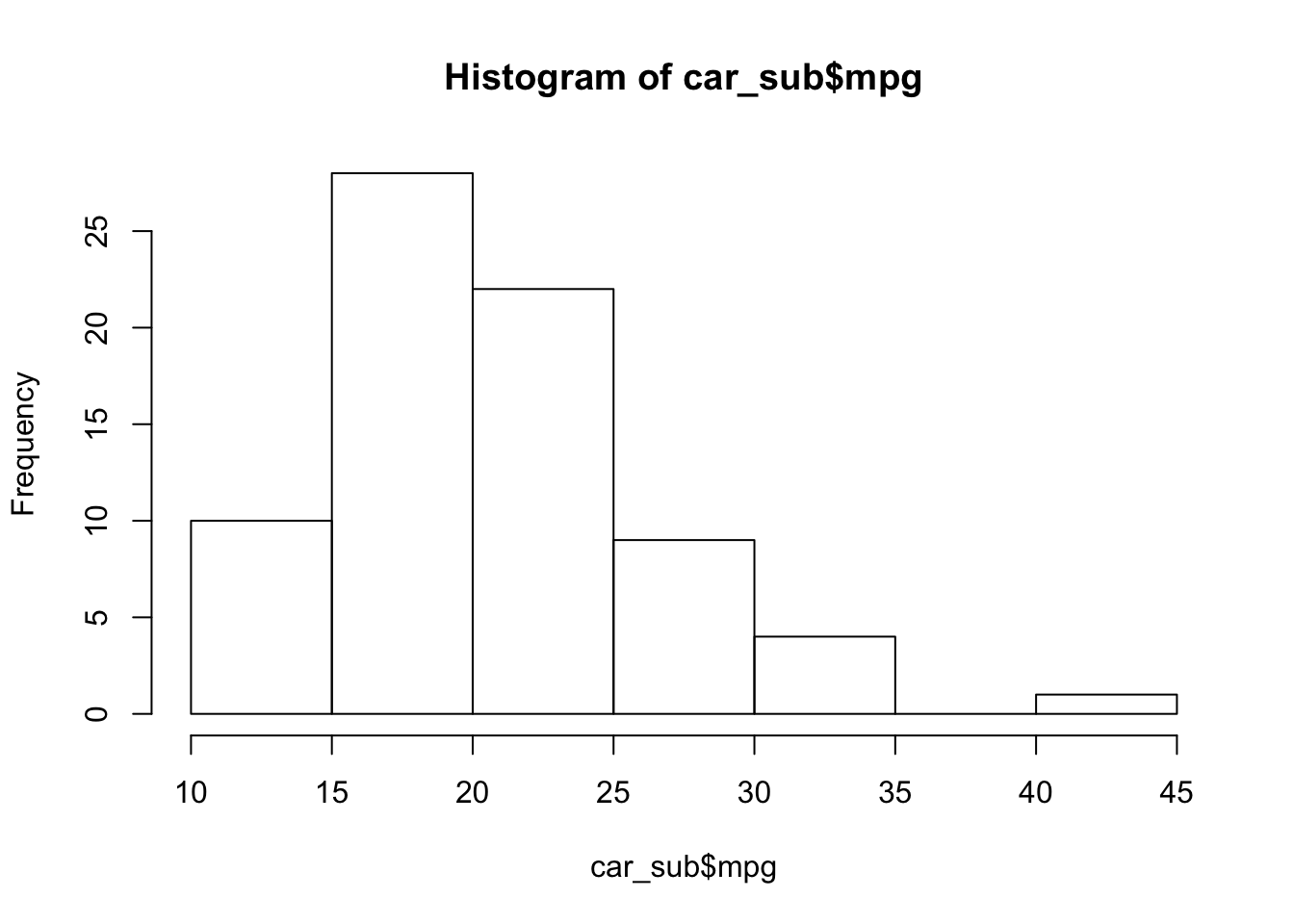Now, a bit prettier. And let’s add a blue line for the median MPG (using the abline() function). # The histogram function hist( # The variable for the histogram x = car_sub$mpg,
# The main title
main = "Distribution of fuel economy",
# The x-axis label
xlab = "MPG (miles per gallon)")
# The blue vertical line at the median MPG (lwd is line width)
abline(v = median(car_sub$mpg), col = "blue", lwd = 3)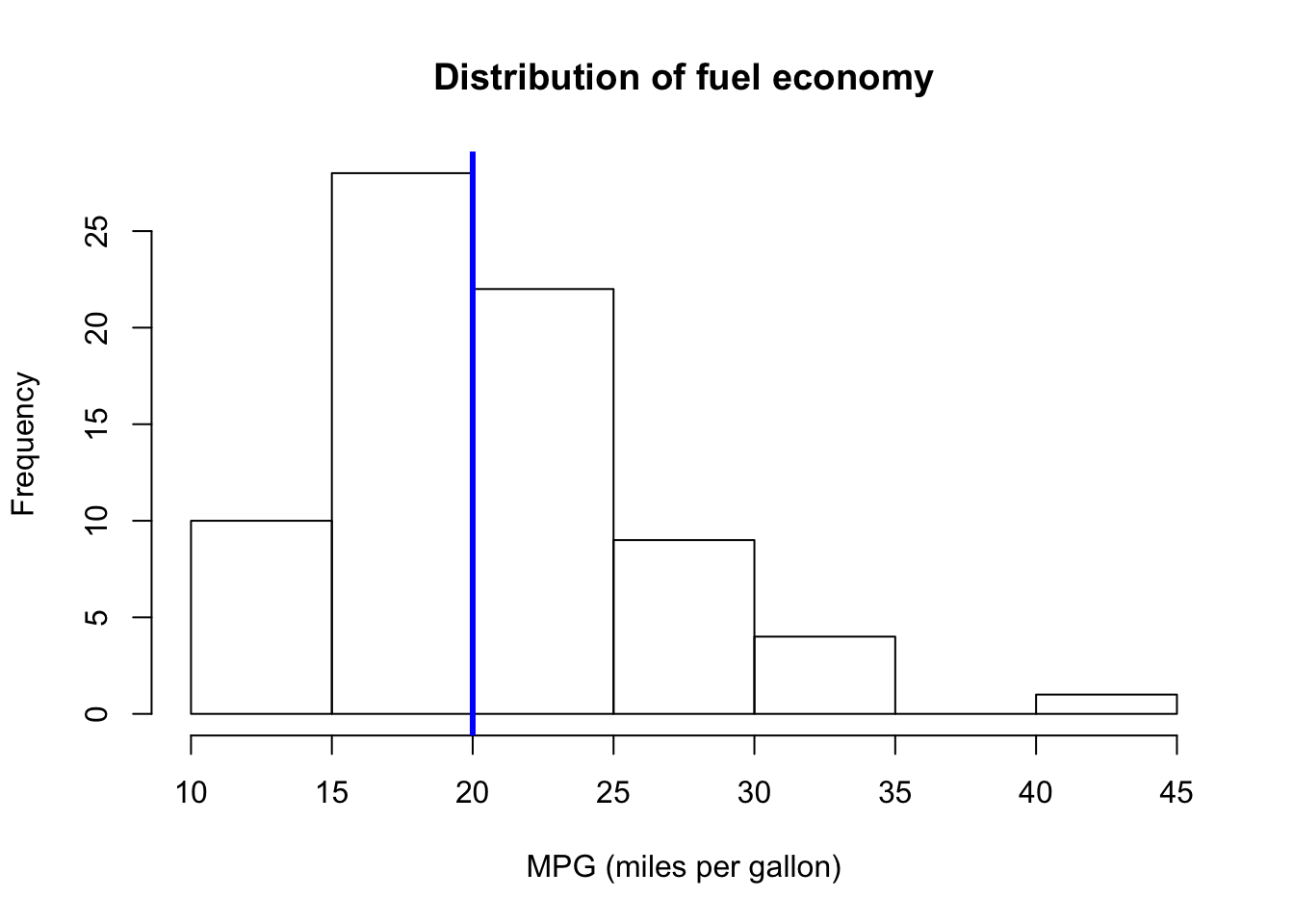Let’s plot price and mileage. A scatterplot will work here, and R’s base plot() function will do just fine with a scatter plot. We will give it an x variable, a y variable, and the axis titles. plot( x = car_sub$mpg,
y = car_sub\$price,
xlab = "Fuel economy (MPG)",
ylab = "Price")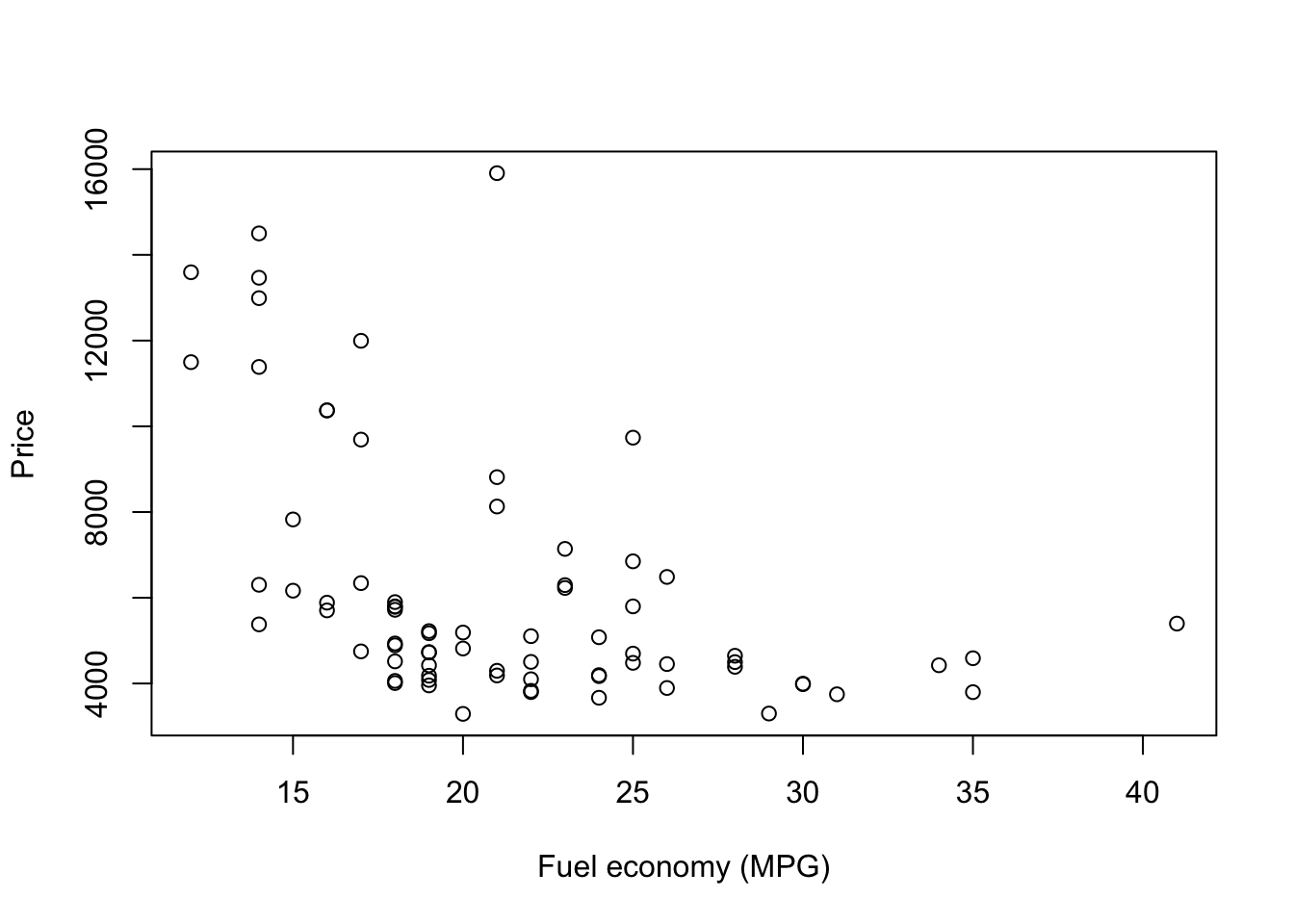Note: I really like clearly defining the arguments of functions. And I recommend it. I find it helps keep things straight, as order matters when you are not naming each argument.

# Indexing

Nearly everything in R is numerically indexed. For instance, when we create a vector of numbers, as we did earlier, each element of the vector gets a numerical index (1, 2, 3, …). You can generally access the individual elements of objects using these indexes and square brackets behind the name of the object (e.g., test grabs that second element of the object test).

# Create a vector
x <- c(3, 5, 7, 9)
# Grab the second element of x
x
##  5
# Grab the second and third elements of x
x[c(2, 3)]
##  5 7
# Grab the second and third elements of x
x[2:3]
##  5 7
# See what 2:3 does
2:3
##  2 3

This indexing works with data objects, as well. We just have one more dimension to consider—we have rows and columns. Rows before columns, i.e., [row, column] (but we don’t actually use the words).

To grab the first row of car_sub, we put a 1 for the row index and leave the column blank.

car_sub[1, ]
## # A tibble: 1 x 4
##   price   mpg weight length
##   <dbl> <dbl>  <dbl>  <dbl>
## 1 4099.   22.  2930.   186.

To grab the first column of car_sub, we

car_sub[, 1]
## # A tibble: 74 x 1
##     price
##     <dbl>
##  1  4099.
##  2  4749.
##  3  3799.
##  4  4816.
##  5  7827.
##  6  5788.
##  7  4453.
##  8  5189.
##  9 10372.
## 10  4082.
## # ... with 64 more rows

You can also use the name of a column as its index

car_sub[, "price"]
## # A tibble: 74 x 1
##     price
##     <dbl>
##  1  4099.
##  2  4749.
##  3  3799.
##  4  4816.
##  5  7827.
##  6  5788.
##  7  4453.
##  8  5189.
##  9 10372.
## 10  4082.
## # ... with 64 more rows

We’ll do a lot more of this indexing stuff in the future.

# Fun challenge

What happens if you give the head() or tail() functions an n that is negative? Can you replicate the behavior using indexing? Can you replicate the behavior of tail() using only the function head()?

# Linear algebra puzzles

Some classic10 R-meets-linear algebra puzzles for your enjoyment. They may use some R concepts that we have not yet covered.

1. Let I5 be a 5 $$\times$$ 5 identity matrix. Demonstrate that I5 is symmetric and idempotent using simple functions in R.
2. Generate a 2 $$\times$$ 2 idempotent matrix X, where X is not the identity matrix. Demonstrate that X = XX.
3. Generate two random variables, x and e, of dimension n = 100 such that x, e ∼ N(0, 1). Generate a random variable y according to the data generating process $$y_i = x_i + e_i$$. Show that if you regress y on x using the canned linear regression routine lm(), then you will get an estimate of the intercept $$\beta_0$$ and the coefficient on x, $$\beta_1$$, such that $$\beta_0 = 0$$ and $$\beta_1 = 1$$.
4. Show that if $$\lambda_1, \lambda_2, \ldots, \lambda_5$$ are the eigenvectors of a 5 $$\times$$ 5 matrix A, then tr(A) = $$\sum_{i=1}^5 \lambda_i$$.

1. If you do not have the most recent versions of R (3.4.3, a.k.a. Kite-Eating Tree) and RStudio (1.1.383), then please check out the directions in Section 0.

2. CRAN stands for [the] Comprehensive R Archive Network

3. You can also access functions within a package without loading the whole package. Let’s say we want to load the happy() function from the fake package without loading the whole fake package. Just type fake::happy(). The double colon :: is the key here. (Note: Not all functions from a package can be accessed this way.) This method also works well when packages overlap in the names that they use for functions.

4. Windows users beware: when you copy the path from File Explorer, the slashes between folders may be in the wrong direction for R: you will need to either change the direction (from \ to /) or double them (from / to //).

5. Note: Mac (OSX) directories tend to start with “/Users/”, while Windows paths start with the name of the drive, for instance, “C:/”. If you’re using Linux, you probably don’t need my help here.

6. The files are apparently classic Stata tutorial files.

7. If you have really big delimited files (csv, tsv, fixed, width, etc.), I recommend the fread() (fast read) function from the data.table package. The whole data.table package is awesome and fast—it’s just a bit less beginner friendly than dplyr.

8. If leaving off the quotation marks makes you uncomfortable—or is actually inhibiting your programming—each dplyr function has a clone that uses standard evaluation. These standard evaluation clones have the same names as their counterparts but with an added underscore (_) at their ends (e.g., select_()).

9. If you are more comfortable with British English, you will be happy to know you can use the functions summarise() and summarise_each().

10. By classic, I mean they’ve shown up in this class’s section notes for several years.